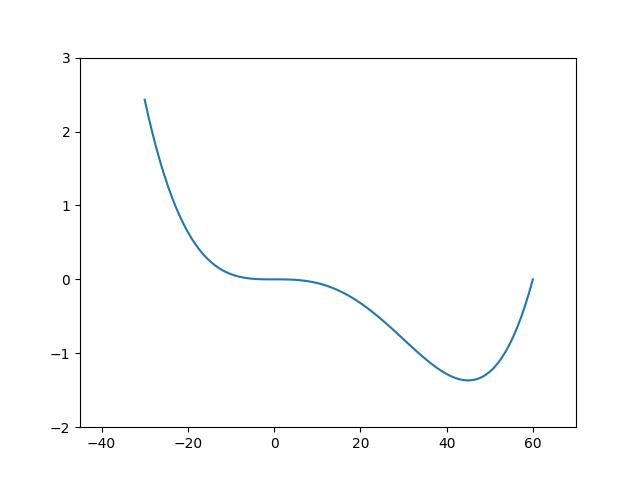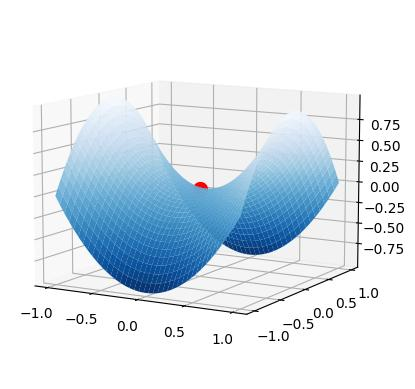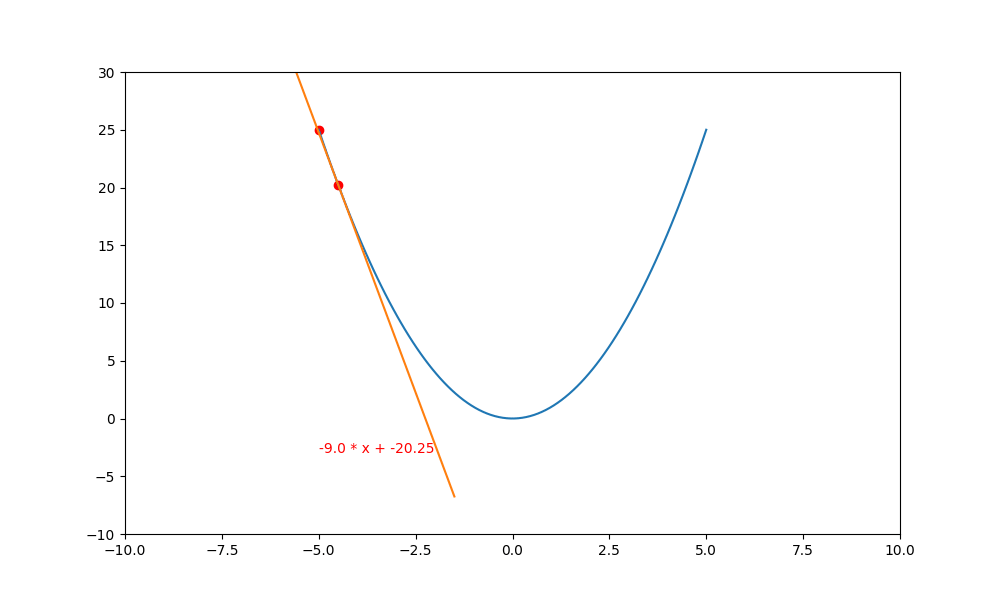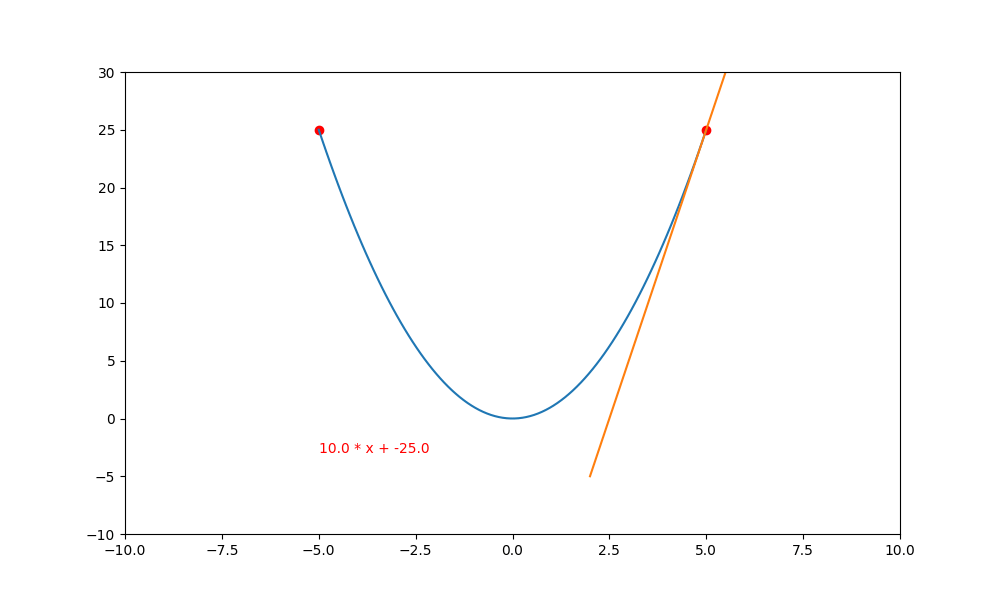# 梯度下降的目的

import numpy as np
import pylab as plt
shrink_y=1e6
ax=plt.subplot()
x=np.linspace(-30,60,100)
y=(np.power(x,4)-60*pow(x,3)-x+1)/shrink_y
ax.set_xlim(-45, 70)
ax.set_ylim(-2, 3)
plt.plot(x, y)
plt.show()# 3D鞍點

from mpl_toolkits.mplot3d import Axes3D
import matplotlib.pyplot as plt
import numpy as np
fig = plt.figure()
x, y = np.mgrid[-1:1:40j, -1:1:40j]
print(x)
print(y)
z = x**2 - y**2
#plot_args不用設定也沒關係，只是設定後圖形比較漂亮
plot_args = dict(
cmap = "Blues_r",
linewidth= 0.4,
alpha =1,
vmin = -1,
vmax = 1)
ax.plot(, , , 'ro', markersize= 10)
ax.plot_surface(x, y, z, **plot_args)
ax.view_init(azim=-60, elev=10)
plt.show()# 批次梯度下降法(Batch Gradient Descent : BGD)

BGD 是最基本的梯度下降法，一般是使用 $x_{t+1} = x_{t} – f'(x_t)*\bigtriangledown x$ 來逼近，$f'(x_t)$ 是 y  函數在 $x_t$ 時的微分(斜率)。 $\bigtriangledown x$ 的值非常小，通常會是在 $1*10^{-6}$ 左右。

import numpy as np
import matplotlib.pyplot as plt

#目標函數 y=x^2
def f(x):
#注意，不可以寫成 return x**2
return np.square(x)+2

#目標函數的一階導數 dy/dx=2*x
def df(x):
return 2 * x

def bias(a,x):
#斜率為 y = ax + b, 此函數在 x 時，值為 f(x)
#所以 ax + b = f(x), 因此 b = f(x) - ax
b=f(x) - a * x
return b

epochs = 80
lr = 0.2
fig=plt.figure(figsize=(9,6))
ax=fig.subplots()

x = np.linspace(-5, 5, 100)
current_x=-5
y=f(x)
traces=[current_x]
for i in range(epochs):
ax.clear()
ax.set_xlim(-10,10)
ax.set_ylim(-2, 35)
plt.plot(x, y, c='b')
plt.scatter(traces, f(traces), c='r')

#對目標函數進行微分
a=df(current_x)
b=bias(a, current_x)

#畫導線
x_l=current_x-3
x_r=current_x+3
line_x=[x_l, x_r]
line_y = [a * (x_l) + b, a * (x_r) + b]
plt.plot(line_x, line_y, c='orange')
ax.text(-2,0, f'y={a:.7f} * x + {b:.7f}', color='red')

#計算下一步 (x,y)
current_x = current_x - a * lr
traces.append(current_x)
plt.pause(0.01)
plt.show()# Tensorflosw 版

import numpy as np
import matplotlib.pyplot as plt
import tensorflow as tf

#目標函數 y=x^2
def f(x):
return np.square(x)+2

#計算偏移量 b 值
def bias(a,x):
b=f(x)- a * x
return b

epochs = 100
lr = 0.2
ax=plt.subplot()
x = tf.linspace(-5, 5, 100)
current_x=tf.Variable(-5.)#x不可以使用 tf.constant, 一定要用 Variable
traces_x=[current_x]
for i in range(epochs):
s = tf.pow(current_x, 2)
ax.clear()
ax.set_xlim(-10,10)
ax.set_ylim(0, 35)
plt.plot(x, f(x))
plt.scatter(traces_x, f(traces_x), c='r')

#進行微分
b=bias(a, current_x)

#繪製導線
x_l=current_x-3
x_r=current_x+3
line_x=[x_l, x_r]
line_y = [a * (x_l) + b, a * (x_r) + b]
plt.plot(line_x, line_y)
ax.text(-5, -15, f'{a} * x + {b}', color='red')
plt.pause(0.01)

#計算下一步 (x, y)
current_x = tf.Variable(current_x - a * lr)
traces_x.append(current_x)
plt.show()


# 放大學習率

..........
xs = np.linspace(-5, 5, 100)
ys = f(xs)
epochs = 200
lr = 0.95
ax=plt.subplot()